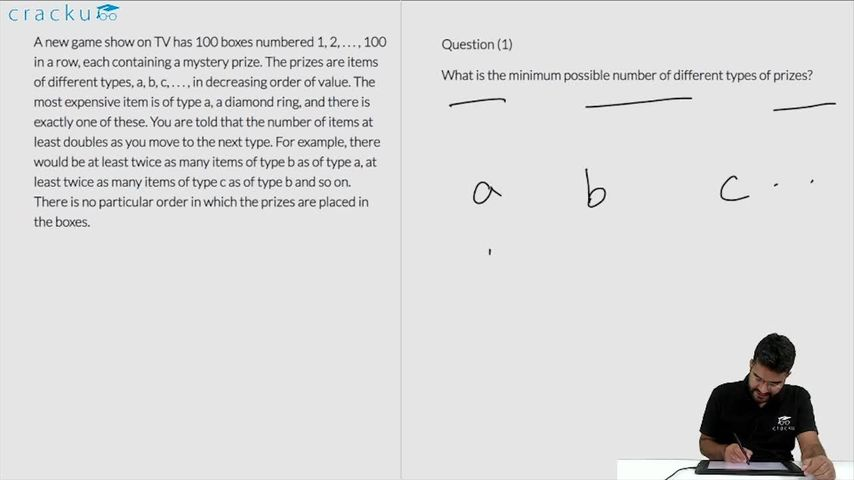### CAT 2019 Question Paper (Slot 1) Question 65

Instructions

A new game show on TV has 100 boxes numbered 1, 2, . . . , 100 in a row, each containing a mystery prize. The prizes are items of different types, a, b, c, . . . , in decreasing order of value. The most expensive item is of type a, a diamond ring, and there is exactly one of these. You are told that the number of items at least doubles as you move to the next type. For example, there would be at least twice as many items of type b as of type a, at least twice as many items of type c as of type b and so on. There is no particular order in which the prizes are placed in the boxes.

Question 65

# Which of the following is not possible?

Solution

Option A: There are exactly 75 items of type e.

a=1,b=2,c=4,d=8, e=85. Here the maximum value of e= 85. Hence it can take the value 75.

An example of such case is a=1,b=2,c=4,d=18, e=75

Option B: There are exactly 30 items of type b.

a=1 b=30 and c=69. Hence this case is also possible.

Option C: There are exactly 45 items of type c.

Since the value of d should be at least 90, it means that d is not present because 45+90 will be more than 100(maximum number of items). Only a,b and c are present.

The maximum value of b = 22 and a =1, but 45+22+1=68, which is less than 100. So this case is not possible.

Option D: There are exactly 60 items of type d.

d=60, c=30, b=9 and a=1. a+b+c+d=100. Hence this case is possible.

### View Video Solution